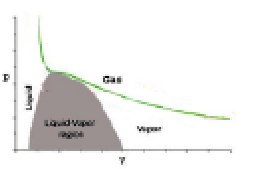Q

# Give answer! Which of following statement (s) is trueI – Slope of isotherm at critical point is maximum.II – Larger is the value of TC easier is the liquification of gas.III – Vander Waal'sequation of state is applicable below c

Which of following statement (s) is true

I – Slope of isotherm at critical point is maximum.

II – Larger is the value of TC easier is the liquification of gas.

III – Vander Waal's equation of state is applicable below critical temperature at all pressure.

• Option 1)

Only I

• Option 2)

I & II

• Option 3)

II & III

• Option 4)

Only II

405 Views

As we learnt in

Critical Temperature -

that temperature upto which gas has to be cooled before gas can be converted into liquid by applying pressure.

-I-    Slope of isotherm below critical point < 0.

Slope of isotherm above critical point < 0.

Slope of isotherm at critical point = 0.

So slope of isotherm at critical point is maximum.

II-

Larger value of TC It means less decreases in temperature is required to liquify the gas. Gas will liquify at higher temperature. So, easier'll be liquification.

III-     When gas is below critical temperature. It is 'liquid' so Vander Waal's equation of state is not valid.

Option 1)

Only I

Incorrect

Option 2)

I & II

Correct

Option 3)

II & III

Incorrect

Option 4)

Only II

Incorrect

Exams
Articles
Questions